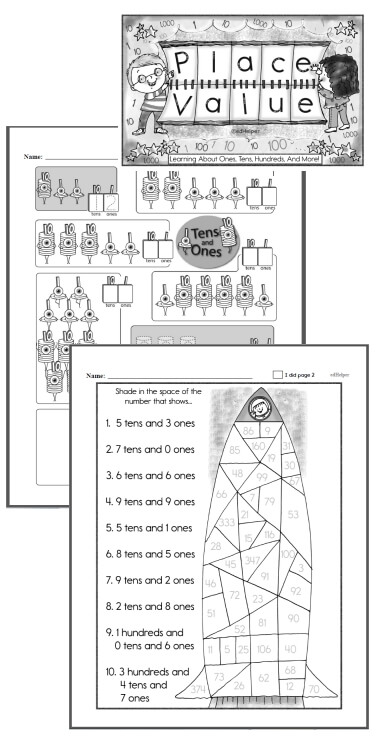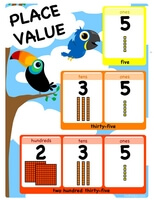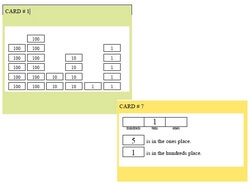Worksheets and No Prep Teaching Resources
Math Worksheets
Place Value Worksheets

# Place Value Math Learning CentersWorksheets, Lessons, and Printables

Place Value No Prep Book #1
Place Value No Prep Book #1 - Not BoringKindergarten - No Prep Place Value Book First Grade - No Prep Place Value Book Second Grade - No Prep Place Value Book

Place Value Math Center - Ones, Tens, and HundredsEasier: Place value words are given under boxes (first answer given) (Grades 1-3) Easier: Place value words are given under boxes (Grades 1-3) Ones, tens, and hundreds place value math learning center (first answer given) (Grades 1-3) Ones, tens, and hundreds place value math learning center (Grades 1-3)

Place Value Math Center - Ones, Tens, Hundreds, and ThousandsEasier: Place value words are given under boxes (first answer given) (Grades 1-3) Easier: Place value words are given under boxes (Grades 1-3) Ones, tens, hundreds, and thousands place value math learning center (first answer given) (Grades 1-3) Ones, tens, hundreds, and thousands place value math learning center (Grades 1-3)

Place Value Math Center - Ones, Tens, Hundreds, Thousands, and Ten-ThousandsEasier: Place value words are given under boxes (first answer given) (Grades 1-3) Easier: Place value words are given under boxes (Grades 1-3) Ones, tens, hundreds, thousands, and ten-thousands place value math learning center (first answer given) (Grades 1-3) Ones, tens, hundreds, thousands, and ten-thousands place value math learning center (Grades 1-3)

Place Value

Have a suggestion or would like to leave feedback?